# How to Compare Two Ranges with VBA in Excel

This post will guide you how to compare two ranges in one worksheet with VBA Macro code and highlight the cells that are different. How do I compare two ranges in two worksheets Using VBA in Excel.

## Compare Two Ranges with VBA

Assuming that you have two lists of data in two worksheets, and you want to compare those two ranges in different two worksheets and find out non-matching cells and then highlight it. How to do it. You can try to use an Excel VBA Macro code to compare two selected ranges in two worksheets.  Here are the steps:

#1 open your excel workbook and then click on “Visual Basic” command under DEVELOPER Tab, or just press “ALT+F11” shortcut.#2 then the “Visual Basic Editor” window will appear.

#3 click “Insert” ->”Module” to create a new module.#4 paste the below VBA code  into the code window. Then clicking “Save” button.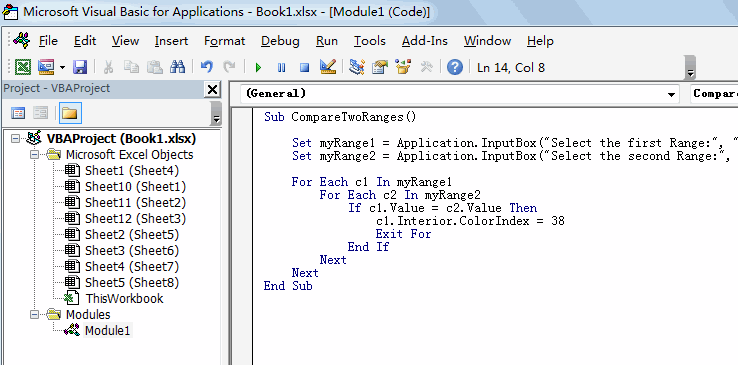```Sub CompareTwoRanges()

Set myRange1 = Application.InputBox("Select the first Range:", "CompareTwoRanges", "", Type:=8)
Set myRange2 = Application.InputBox("Select the second Range:", "CompareTwoRanges", Type:=8)

For Each c1 In myRange1
For Each c2 In myRange2
If c1.Value = c2.Value Then
c1.Interior.ColorIndex = 38
Exit For
End If
Next
Next
End Sub```

#5 back to the current worksheet, then run the above excel macro. Click Run button.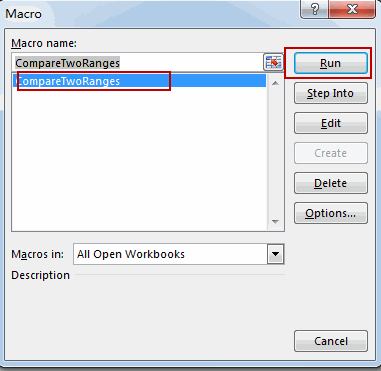#6 Select the first range in Sheet2 worksheet. Click Ok button.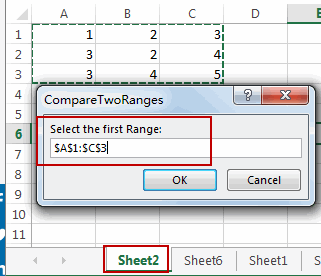#7 Select the second range in Sheet6 worksheet. Click Ok button.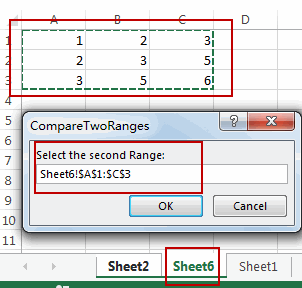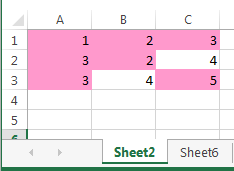You should notice that all duplicate values have been highlighted with background color you set.

Sidebar